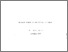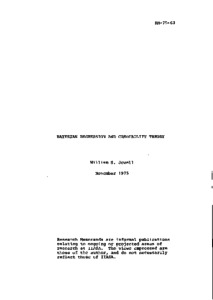Bayesian Regression and Credibility Theory

Jewell, W.S. (1975). Bayesian Regression and Credibility Theory. IIASA Research Memorandum. IIASA, Laxenburg, Austria: RM-75-063Preview
Text
RM-75-063.pdf

Abstract

The development of a Bayesian theory of regression requires special distributional assumptions and rather complicated calculations. In this paper, general formulae for predicting the mean values of the regression coefficients and the mean outcomes of future experiments are developed using the methods of credibility theory, a linearized Bayesian analysis originally used in actuarial problems. No special distributional assumptions on prior or error distributions are needed, and heteroscedastic errors in both the dependent and independent variables are permitted. The first group of formulae hold for arbitrary design matrices and dimensionality of input, since, as common in Bayesian methods, there are none of the usual problems of identifiability. However, in the event that the design matrix has full rank, the credibility results are equivalent to a linear mixture of the prior mean prediction and the classical (generalized) least-squares regression predictor; thus, the credibility result provides a bridge between full Bayesian methods and classical estimators. One can also find easily the preposterior covariance matrix for the credibility estimators, and it is shown that prior information and the results from prior experiments can be cascaded in a particularly intuitive manner. Many special applications of the credibility formulae are possible because of the generality of the assumptions.

Item Type: Monograph (IIASA Research Memorandum) System and Decision Sciences - Core (SDS) IIASA Import 15 Jan 2016 01:42 27 Aug 2021 17:08 http://pure.iiasa.ac.at/453View Item